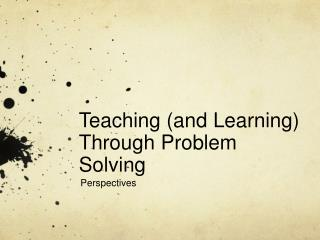Download PresentationTeaching (and Learning) Through Problem SolvingTeaching (and Learning) Through Problem Solving - PowerPoint PPT Presentation

Download PresentationTeaching (and Learning) Through Problem Solving
An Image/Link below is provided (as is) to download presentation

Download Policy: Content on the Website is provided to you AS IS for your information and personal use and may not be sold / licensed / shared on other websites without getting consent from its author. While downloading, if for some reason you are not able to download a presentation, the publisher may have deleted the file from their server.

- - - - - - - - - - - - - - - - - - - - - - - - - - - E N D - - - - - - - - - - - - - - - - - - - - - - - - - - -
Presentation Transcript

1. Teaching (and Learning) Through Problem Solving Perspectives

2. TTPS: Teaching ABOUT Problem Solving • For the last 25-30 years, “Problem Solving” has become an increasing focus of mathematics education, including elementary level mathematics. • Teaching About Problem Solving has been the focus for much of that time. What does this mean? • Briefly: • Teaching students specific strategies to use in PS situations • Providing a general model as a guide to follow when engaging in problem solving

3. TTPS: Teaching ABOUT Problem Solving • The Ontario Ministry of Education (Gr. 1-8 Math doc) • The Four-Step Problem Solving Model (after Polya) • Understand the Problem • Make a Plan • Carry Out the Plan • Look Back at the Solution • Infused throughout: “Communication” (p. 13) • This Model should be thought of as dynamic, not static • Textbook author Small (ch. 3/ch. 5—2nd ed.) • Refers to this same model as “The Inquiry Process”

4. TTPS: Teaching ABOUT Problem Solving • The emphasis in Teaching About Problem Solving: • On providing students with knowledge of strategies that they can then apply in PS situations. Small (2013) describes several PS strategies in chapter 5 • Students then have strategy tools which they can apply while they work through the four-stage model • A concern has been that problem solving often is applied in an “after teaching the content” mode. • A linear sequential process. • Strategy choice has largely been pre-established

5. Teaching THROUGH Problem Solving • More recent: Teaching THROUGH problem solving • Small (2013) notes, in Chapter 5: • “To teach through problem solving, the teacher provides a context or reason for the learning by focusing instruction on a problem to be solved and, within that context and based on student work, drawing out any procedures” (p. 96). • Van de Walle & Folk (2008) note: • “Problem-based tasks or activities are the vehicle by which the desired curriculum is developed. The learning is an outcome of the problem-solving process” (p. 38).

6. Teaching THROUGH Problem Solving • The OME (2005) says of learning through problem solving: • Problem solving is central to learning mathematics. By learning to solve problems and by learning through problem solving, students are given numerous opportunities to connect mathematical ideas and to develop conceptual understanding. Problem solving forms the basis of effective mathematics programs and should be the mainstay of mathematical instruction. (p. 11) • Strategies can still be discussed, as they are not all naturally occurring.

7. Teaching THROUGH Problem Solving • A common format for TTPS is the three-phase lesson plan: Before, During, and After (Van de Walle & Folk, 2008) • Before: (“Getting Ready”) • Prepare the Students and Provide the Problem • Be certain the problem is understood • Activate useful prior knowledge • Establish clear expectations for products

8. Teaching THROUGH Problem Solving • During: (“Students Work”) • Listen carefully • Provide appropriate hints • Observe and assess • Hand over ownership to the students during this process • After: (“Class Discussion”) • Encourage a community of learners • Listen, and accept student solutions w/o evaluation • Summarize main ideas, identify future problems

9. Teaching THROUGH Problem Solving • Consider the Problem we recently solved collaboratively: • How might the three phases of the lesson be applied to that classroom experience? • Then let’s try another problem and develop a plan • Chapter 2 Problem, Small (2008 & 2013). P. 17. • 30 minutes to develop; 30 minutes to share

10. Teaching THROUGH Problem Solving • Here is the Problem at the start of Chapter 2 (Small, 2013): • Imagine using 4 of the 12 long tick marks on a round [analogue] clock face as vertices . . . Of rectangles. • [ticks signifying hours] • How many different rectangles could you create? • How would the answer change if you used four of the 60 tick marks? • [ticks signifying minutes] • How would you get this started?

11. Teaching THROUGH Problem Solving • For an example of the Schulich School of Education lesson plan adapted to the “three-phase” lesson, see the Grade 7 sample, “Finding the General Formula for Area of a Trapezoid” in the Practice Teaching Handbook. • In 2012-13 this is Lesson Plan Sample 4 found on p. 46